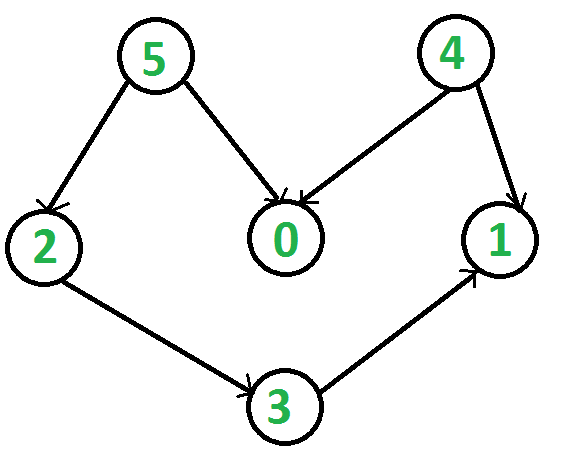# Python Program for Topological Sorting

• Difficulty Level : Hard
• Last Updated : 10 Aug, 2021

Topological sorting for Directed Acyclic Graph (DAG) is a linear ordering of vertices such that for every directed edge uv, vertex u comes before v in the ordering. Topological Sorting for a graph is not possible if the graph is not a DAG.
For example, a topological sorting of the following graph is “5 4 2 3 1 0”. There can be more than one topological sorting for a graph. For example, another topological sorting of the following graph is “4 5 2 3 1 0”. The first vertex in topological sorting is always a vertex with in-degree as 0 (a vertex with no in-coming edges).Topological sorting can be implemented recursively and non-recursively. First, we show the clearer recursive version, then provide the non-recursive version with analysis.

## Python

 `#Python program to print topological sorting of a DAG``from` `collections ``import` `defaultdict` `#Class to represent a graph``class` `Graph:``    ``def` `__init__(``self``,vertices):``        ``self``.graph ``=` `defaultdict(``list``) ``#dictionary containing adjacency List``        ``self``.V ``=` `vertices ``#No. of vertices` `    ``# function to add an edge to graph``    ``def` `addEdge(``self``,u,v):``        ``self``.graph[u].append(v)` `    ``# A recursive function used by topologicalSort``    ``def` `topologicalSortUtil(``self``,v,visited,stack):` `        ``# Mark the current node as visited.``        ``visited[v] ``=` `True` `        ``# Recur for all the vertices adjacent to this vertex``        ``for` `i ``in` `self``.graph[v]:``            ``if` `visited[i] ``=``=` `False``:``                ``self``.topologicalSortUtil(i,visited,stack)` `        ``# Push current vertex to stack which stores result``        ``stack.insert(``0``,v)` `    ``# The function to do Topological Sort. It uses recursive``    ``# topologicalSortUtil()``    ``def` `topologicalSort(``self``):``        ``# Mark all the vertices as not visited``        ``visited ``=` `[``False``]``*``self``.V``        ``stack ``=``[]` `        ``# Call the recursive helper function to store Topological``        ``# Sort starting from all vertices one by one``        ``for` `i ``in` `range``(``self``.V):``            ``if` `visited[i] ``=``=` `False``:``                ``self``.topologicalSortUtil(i,visited,stack)` `        ``# Print contents of stack``        ``print` `stack` `g``=` `Graph(``6``)``g.addEdge(``5``, ``2``);``g.addEdge(``5``, ``0``);``g.addEdge(``4``, ``0``);``g.addEdge(``4``, ``1``);``g.addEdge(``2``, ``3``);``g.addEdge(``3``, ``1``);` `print` `"Following is a Topological Sort of the given graph"``g.topologicalSort()``#This code is contributed by Neelam Yadav`

Output:

```Following is a Topological Sort of the given graph
5 4 2 3 1 0```

## Non-recursive Topological Sorting

### Algorithm:

The way topological sorting is solved is by processing a node after all of its children are processed. Each time a node is processed, it is pushed into a stack in order to save the final result. This non-recursive solution builds on the same concept of DFS with a little tweak which can be understood above and in this article. However, unlike the recursive solution, which saves the order of the nodes in the stack after all the neighboring elements have been pushed to the program stack, this solution replaces the program stack with a working stack. If a node has a neighbor that has not been visited, the current node and the neighbor are pushed to the working stack to be processed until there are no more neighbors available to be visited.
After all the nodes have been visited, what remains is the final result which is found by printing the stack result in reverse.

## Python

 `#Python program to print topological sorting of a DAG``from` `collections ``import` `defaultdict` `#Class to represent a graph``class` `Graph:``    ``def` `__init__(``self``,vertices):``        ``self``.graph ``=` `defaultdict(``list``) ``#dictionary containing adjacency List``        ``self``.V ``=` `vertices ``#No. of vertices` `    ``# function to add an edge to graph``    ``def` `addEdge(``self``,u,v):``        ``self``.graph[u].append(v)` `    ``# neighbors lazy generator given key``    ``def` `neighbor_gen(``self``,v):``        ``for` `k ``in` `self``.graph[v]:``            ``yield` `k``    ` `    ``# non recursive topological sort``    ``def` `nonRecursiveTopologicalSortUtil(``self``, v, visited,stack):``        ` `        ``# working stack contains key and the corresponding current generator``        ``working_stack ``=` `[(v,``self``.neighbor_gen(v))]``        ` `        ``while` `len``(working_stack) > ``0``:``            ``# get last element in stack``            ``v, gen ``=` `working_stack[``-``1``]``            ``visited[v] ``=` `True``            ` `            ``# delete it from stack``            ``working_stack.pop()``            ` `            ``# run through neighbor generator until its empty``            ``continue_flag ``=` `True``            ``while` `continue_flag:``                ``next_neighbor ``=` `next``(gen,``None``)``                ` `                ``# if generator has returned all neighbors``                ``if` `next_neighbor ``is` `None``:``                    ``continue_flag ``=` `False``                    ``# Save current key into the result stack``                    ``stack.append(v)``                    ``continue``                ` `                ``# if new neighbor push current key and neighbor into stack``                ``if` `not``(visited[next_neighbor]):``                    ``working_stack.append((v,gen))``                    ``working_stack.append((next_neighbor,``self``.neighbor_gen(next_neighbor)))``                    ``continue_flag ``=` `False``            ` `    ``# The function to do Topological Sort.``    ``def` `nonRecursiveTopologicalSort(``self``):``        ``# Mark all the vertices as not visited``        ``visited ``=` `[``False``]``*``self``.V``        ` `        ``# result stack``        ``stack ``=` `[]` `        ``# Call the helper function to store Topological``        ``# Sort starting from all vertices one by one``        ``for` `i ``in` `range``(``self``.V):``            ``if` `not``(visited[i]):``                ``self``.nonRecursiveTopologicalSortUtil(i, visited,stack)``         ``# Print contents of the stack in reverse``        ``print``(stack[::``-``1``])` `g``=` `Graph(``6``)``g.addEdge(``5``, ``2``);``g.addEdge(``5``, ``0``);``g.addEdge(``4``, ``0``);``g.addEdge(``4``, ``1``);``g.addEdge(``2``, ``3``);``g.addEdge(``3``, ``1``);` `print``(``"The following is a Topological Sort of the given graph"``)``g.nonRecursiveTopologicalSort()``# The following code was based of of Neelam Yadav's code and is modified by Suhail Alnahari`

Output:

```Following is a Topological Sort of the given graph
5 4 2 3 1 0```

### Complexity Analysis:

• Time Complexity: O(V + E): The above algorithm is simply DFS with a working stack and a result stack. Unlike the recursive solution, recursion depth is not an issue here.
• Auxiliary space: O(V): The extra space is needed for the 2 stacks used.

Please refer complete article on Topological Sorting for more details.

My Personal Notes arrow_drop_up